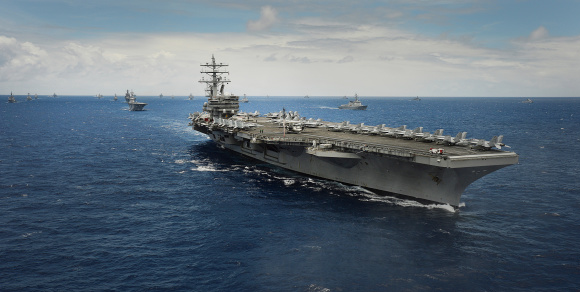## Aircraft Carrier

Imagine fighter jets on an aircraft carrier are launched off a 300 m runway from rest. If the jets’ acceleration is 10 m/s^2, what is the minimum takeoff speed?Hint
$$v^2=v_0^2+2a_0(s-s_0)$$$where $$v$$ is the velocity along the direction of travel, $$v_0$$ is the velocity at time $$t_0$$ , $$a_0$$ is constant acceleration, $$s$$ is the displacement at time $$t$$ along the line of travel, and $$s_0$$ is the displacement at time $$t_0$$ . Hint 2 Solve for $$v$$ . For constant acceleration, the equation for velocity as a function of position: $$v^2=v_0^2+2a_0(s-s_0)$$$
where $$v$$ is the velocity along the direction of travel, $$v_0$$ is the velocity at time $$t_0$$ , $$a_0$$ is constant acceleration, $$s$$ is the displacement at time $$t$$ along the line of travel, and $$s_0$$ is the displacement at time $$t_0$$ . Since the jets takeoff from rest, $$v_0=0$$ :
$$v^2=(0m/s)^2+2(10m/s^2)(300m)$$$$$v=\sqrt{2(10m/s^2)(300m)}=\sqrt{6,000m^2/s^2}=77.46\:m/s$$$
77.46 m/s